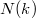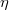﻿

Certain results on$N(k)$-paracontact metric manifolds

Abstract

The purpose of this paper is to study conformally flat$N(k)$-paracontact metric manifolds. Also we study$\eta$-Ricci solitons on three-dimensional$N(k)$-paracontact metric manifolds. Finally, we study gradient Ricci solitons on three-dimensional contractible$N(k)$-paracontact metric manifolds.

DOI Code: 10.1285/i15900932v38n2p21

Keywords: $N(k)$-paracontact; conformally flat; $\eta$-Ricci soliton; gradient Ricci soliton; para-Sasakian manifold

Full Text: PDF